# A Review of transmission lines as circuit elements

Home - Techniek - Electronica - Radiotechniek - Radio amateur bladen - QST - A Review of transmission lines as circuit elements

Many amateurs active on the v.h.f. bands enjoy building their own equipment. The r.f. circuits often consist of hardware or plumbing which involves considerable metal work. Cut-and-try methods involve much more time and expense than at the lower frequencies.

I will review some of the design details involved in high-frequency circuit construction, so that most of the cut-and-try work can be done on paper.

Transmission-line sections are used as circuit elements at v.h.f. because of their desirable impedance properties. Lines that are used for such purposes are usually open-circuited or short-circuited at the receiving end, and do not serve to actually transmit energy. The term "transmission line" is used for purposes of clarity.

### Equivalent circuits

If we consider only what appears at the input terminals, a short-circuited quarter-wavelength line and a parallel-resonant circuit, of coil and capacitor, have these characteristics in common; both present extremely high impedance at one particular frequency; with both, the impedance at resonance is resistive and the impedance drops rapidly if the frequency varies slightly from resonance. Both will carry direct current freely while effectively blocking the frequency to which they are resonant.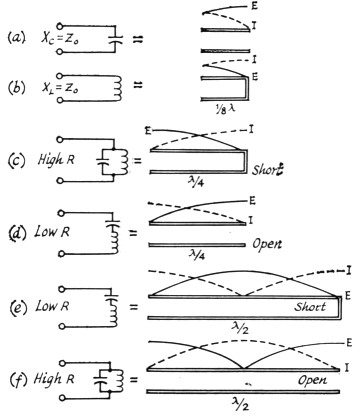Fig. 1. Table of equivalent circuits using resonant lines. Voltage and current relationships are illustrated for open and shorted line.

An inherent difference is that the transmission line displays similar resonance at all odd multiples of its lowest resonant frequency; and has the inverse resonance characteristics of a shorted half-wavelength line at the even multiples.

An open-circuited quarter-wavelength line is similar to a series-resonant circuit of coil and capacitor. It has extremely low impedance at the resonant frequency, is resistive at resonance while being inductive above and capacitive below this frequency. It blocks direct current while freely passing the resonant-frequency r.f. energy. Like a short-circuited line (but unlike a circuit of lumped constants), its characteristics tend to repeat at odd multiples of the lowest resonant frequency, whereas at even multiples the inverse characteristics appear.

An open-circuited half-wavelength line is similar to a short-circuited quarter-wavelength line in that both have the same Q and are thus equally selective in a resonant circuit. However, at radio frequencies other than the desired resonant frequency (such as half and double the fundamental resonant frequency), the open and short-circuited lines have quite different characteristics. This may be important in connection with harmonics.

With a quarter-wave line, the closest resonant frequencies to the fundamental occur at odd multiples such as 3, 5 and 7 times the fundamental frequency. With a half-wave line, they occur at multiples of 2, 3 and 4 times the fundamental. A quarter-wave resonant line, therefore, gives greater separation of the higher-resonant frequencies from the fundamental.

### Parallel lines

Parallel lines are most often used with push-pull circuits, in either quarter-wave or half-wave configuration. With half-wavelength lines, the B plus is connected at the electrical center of the lines, and often a coil, resonant at a lower frequency, is placed here to give multiband operation.

Parallel lines are relatively easy to construct. Their electrical length may be readily changed with short-circuiting bars, and when they are used with appropriate types of tubes, the connections between lines and tube terminals can be short and direct. Furthermore, these connections and the portions of the tube leads inside the envelope become parts of the resonant-line system. For very high frequencies, the tube leads may constitute the principal part of this system but are largely inaccessible for purposes of power-output coupling. In some cases, the portion of the circuit from which power is to be coupled may be operated at a multiple length of the shortest possible line; e.g., three-quarter rather than one-quarter wavelength.

Since open parallel lines radiate electromagnetic energy when excited, it is necessary to shield these lines for optimum performance. The parts, such as sides and covers, of the metal boxes used as the shield should be well bonded together, either with screws or by contact fingers. This is because electromagnetic shielding depends on the flow of induced currents in the metal of the shield. For the same reason, the shield should be constructed from material of high conductivity. For ultra-high frequencies, silver plating is desirable.

Several methods of tuning are available. An adjustable short-circuiting strap can be used, which must make good electrical contact. If the line is also short-circuited at the end by a large disk of copper or other good conducting material, it will be more effective. A butterfly capacitor, or a parallel-plate capacitor, may be placed anywhere along the line the tuning effect becoming less pronounced as the capacitor is located nearer the shorted end of the line.

The characteristic impedance of parallel conductors may be calculated as follows: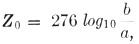where b is the center-to-center spacing of the conductor and a is the radius of the conductors. This relationship is shown in Fig. 2.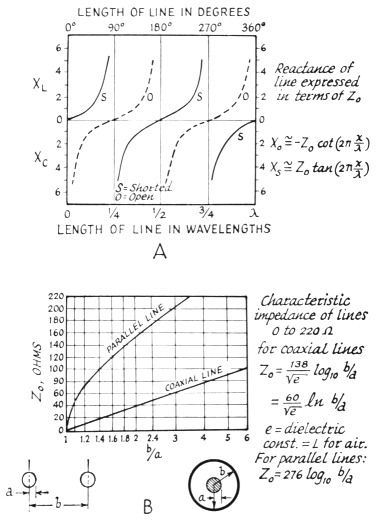Fig. 2. Chart showing reactance of lines, expressed in terms of Z0, is illustrated at A. At B, a chart showing the characteristic impedance of lines from 0-220 ohms.

For two-wire lines, minimum attenuation theoretically will occur when b/a = 2.7. However, when proximity effect is included, the optimum b/a ratio is about 4. The b/a ratio to give maximum impedance to a short-circuited quarter-wavelength 2-wire line is about 8.0.

### Coaxial lines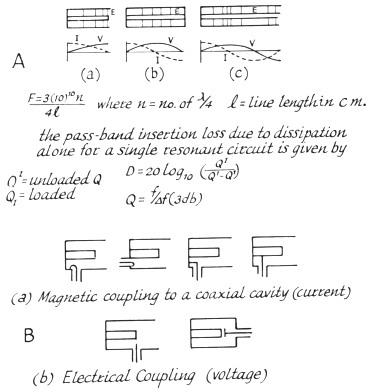Fig. 3. Approximate voltage and current distribution in one-quarter wavelength (A), one-half wavelength (B), and three-quarter wavelength (C) resonant coaxial lines. The field strength, E, is also shown. At B, an illustration of magnetic and electrical coupling to coaxial cavity circuits.

When the various characteristics (Fig. 4) of a coaxial transmission line are considered, such as attenuation, resonant impedance, breakdown voltage, and power-carrying capacity, an optimum ratio of b/a = 3.6 is found to exist, where b is the inner radius of the outer conductor, and a is the outer radius of the inner conductor. Minimum attenuation occurs at this value, which also corresponds to a characteristic impedance of 77 ohms for a line with air dielectric. This is an important reason for the widespread practical use of lines with approximately this impedance.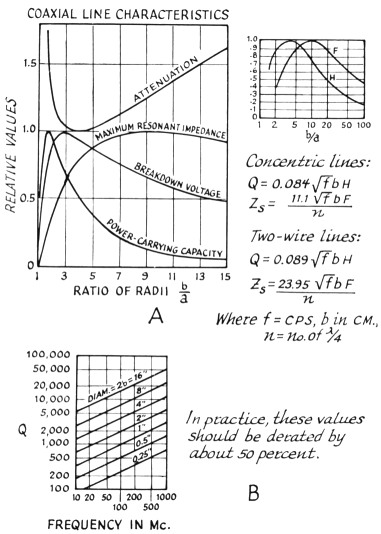Fig. 4. Graphic representations of coaxial line characteristics are shown at A. At 6, a chart showing Q in connection with element diameters and frequency, for concentric lines based on b/a = 3.6, using copper lines and air dielectric.

Physically, if the inner conductor is smaller than the optimum size, its resistance is higher and loss is increased. If the inner conductor is larger than optimum, the increased capacitance lowers the value of Z and hence more current is required to transmit a certain amount of power, with the result that loss is again increased.

However, a line designed for minimum attenuation is not best for all purposes. A line may be designed to transmit maximum power. The limiting factor is electric field strength at the surface of the inner conductor; if a critical value of field strength (about 30,000 volts per centimeter) is exceeded, corona or sparking results. The optimum value of b/a for maximum power transmission is 1.65, and the corresponding characteristic impedance is 30 ohms.

When a line is designed to act as a resonant circuit, other values of b/a may be preferred. For a short-circuited resonant coaxial line to have maximum impedance, b/a should be 9.2, corresponding to Zo equals 133 ohms for an air-insulated line. For an open-circuited resonant line to have minimum impedance, the inner conductor of the coaxial line should be as large as possible, requiring Zo to approach zero.

### Coaxial-line oscillators and amplifiers

The adoption of conventional oscillator and amplifier circuits to u.h.f. use is facilitated by the use of coaxial lines as circuit elements. The high inherent Q of concentric lines as resonant circuits, the very low radiation, and the possibility of isolation of the circuits, contribute to successful design. The lighthouse tube is designed especially for such circuits. The cylindrical, or dish construction, is carried through from the external terminal of the tube to the active part of the tube elements. A high degree of circuit isolation is thus possible, and coupling between circuits is reduced to a minimum.

The grounded-grid circuit is often used for oscillators and amplifiers at u.h.f. and is particularly advantageous in amplifier operation. The feedback or coupling capacitance between output and input circuits is the plate-cathode capacitance, which is reduced to a minimum in most tubes suitable for coaxial circuit use. Thus, regeneration through interelectrode feedback is materially reduced by grid shielding.

The similarity between the grid-separationtype oscillator and amplifier circuits is consider- able. The conversion of an oscillator to an amplifier consists primarily of removing the external feedback system, the addition of a source of driving energy, and retuning. The plate-circuit loaded Q will influence both the frequency stability and modulated bandwidth of an oscillator and, for a given loaded resonant impedance, will depend on line dimensions, tube capacitance, and the operating mode.

Whereas in the ideal case, the expression for the input impedance of the coaxial line is frequently treated as a pure reactance, it should not be forgotten that the line is actually a circuit element with distributed constants, both inductive and capacitive. While the inductive reactance of a short-circuited line less than 90 degrees in length may be used to tune out a terminating capacitive reactance, the total capacitance in the resonant circuit is materially increased by that which is distributed in the line.

The distributed capacitance of a coaxial line is a function of the characteristic impedance. This is of importance where high operating Q must be considered for its limitation on the modulated bandwidth or, in the case of an oscillator, for its influence on frequency stability. A given input reactance might be obtained with a short high-characteristic-impedance line or a long low-characteristic-impedance line. The resonant circuit Q of the short line when shunt-loaded with a given resistance will be lower than that of the longer line if the electrical length of the lines is less than 90 degrees. The extra storage of energy in the low-impedance line will increase its operating Q over that of the high-impedance line. Where physical dimensions are concerned, low and high might be considered to be about 20 and 90 ohms, respectively.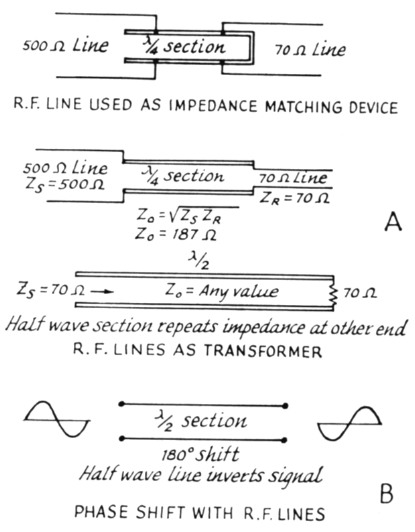Fig. 5. Illustrations of various applications for parallel line sections as discussed in the text, at A. Phase-shift characteristics for line sections are shown at B.

### Limitations on Tuning Range

A practical limitation on the low-frequeucy range of a coaxial oscillator or amplifier is the actual physical length of the line elements, which rapidly increases as the frequency is lowered. This can be appreciated when the actual physical quarter-wavelength is considered at low frequencies, for the resonant lines approach this length quite closely as the reactance of a fixed terminating capacitance increases with the decrease in frequency.

When over-all physical length is an important consideration, it is helpful to remember that a given terminating capacitance may he resonated, with a fixed-maximum length of line, to a lower frequency with a line of higher characteristic impedance.

Physical dimensions also influence the practicable upper-frequency limit of coaxial lines as resonant circuit elements. This results from the ability of cavities of large radial electrical dimensions to support interfering waveguide and spurious coaxial-resonance modes. The principal interfering higher-order coaxial-resonance mode is the TE mode, which can exist only at wavelengths less than the cutoff value given by: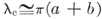where a is the radius of the inner conductor, and b the radius of the outer conductor. In any event, this TE mode should not interfere if the resonant-circuit line lengths are less than 90 degrees.

### Coaxial filters

Preselectors, or handpass filters, are often made using quarter-wave or three-quarter-wave coaxial resonators. These can be nearly identical to coaxial v.h.f. amplifiers except that they are passive circuits. A preselector is a device used to pass discrete bands of frequencies within a limited operating range, while rejecting signals at frequencies outside its passband. It can be very useful in suppressing transmitter harmonics and in reducing receiver overloading due to strong signals outside the amateur v.h.f. bands.

When designing a filter, it is necessary to know the minimum passband attenuation and bandwidth desired. If it is made tunable, then the filter can be adjusted for minimum loss at any particular frequency. Nearly all the characteristics of a coaxial filter can be related to QU and QL, where QU is the unloaded Q of the filter, and QL is the loaded Q of the filter. The unloaded Q of a cavity depends on the frequency and the impedance and size of the cavity. The theoretical QU of a coaxial cavity can be obtained from the equation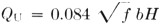where b is in centimeters, f is in c.p.s. and H a factor related to b/a as shown in Fig. 4, at A. The Q of resonant coaxial lines of optimum proportions (b/a = 3.6) is shown in Fig. 4, at B. Usually, these values must be derated from 10 to 50 per cent because of lower conductivity than predicted, contact resistance between movable and fixed parts of a cavity, capacitive loading effects of coupling elements and end plates, and other unavoidable imperfections.

Losses in coaxial filters are of two kinds - mismatch and dissipation. If the filter is simply inserted in a 50- or 70-ohm line, a good match can be obtained if the input and output loops have the same size and shape and are located at points of equal intensity. Usually, the effect of self-inductance of the coupling loops is merely to shift the resonant frequency slightly.

Dissipation (or resistive) loss is an important factor in narrow-band filters because of the relatively high values of QL required for narrow passbands.

The passband insertion loss, due to dissipation alone, for a single resonant circuit is given by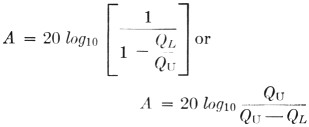where A is the dissipative loss in dB. To have an insertion loss of less than 1 dB, QU must equal 10 QL.

The Q of a resonant circuit may also be defined as the ratio of the mean passband frequency to the 3-dB bandwidth (F / f or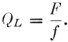Since selectivity and insertion loss are directly related to QL, both functions can be adjusted for any particular need by making the coupling variable (such as rotatable loops).

If two or more cavities are used in series to increase the selectivity, they should be spaced an electrical one-quarter wavelength from center to center.

The position of the loops, with respect to the center conductor of the cavity, also has an effect on QL. The closer the coupling the lower the QL and the greater the bandwidth.

In practice, a certain amount of electrical coupling will be combined with the magnetic coupling of the loop, depending on the size of the loop.

As an example, a coaxial filter for two meters might be designed to cover the entire band of 4 megacycles. Thus,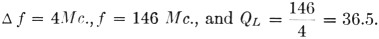To keep the insertion loss A below 1 db., QU should be 365. From Fig. 4B, a coaxial cavity of -inch outer diameter has a theoretical Q of about 600. Usually, more selectivity than this is desired, and a previous article listed typical cavity dimensions for the various v.h.f. bands.(1)

A filter such as this can be made tunable either by changing the length of the inner conductor or by capacitive loading. The latter is generally less difficult to accomplish.

The best method in constructing transmitters, converters or filters using resonant line elements is to follow the ideas in articles in the handbooks and magazines. A typical circuit for parallel-line construction is the 2-meter transmitter de-1 scribed in QST.(2) A coaxial-line amplifier for 2 meters is described in an earlier issue of QST.(3) An important consideration, when constructing similar equipment, is to determine the length of the quarter-wave section of transmission line. k The equation used to solve this problem is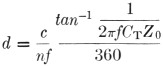where
d = quarter-wave resonant length in inches.
c = velocity of propagation in a vacuum (1.18 × 1010 inches/sec.).
n = index of refraction of the dielectric medium = 1 for air.
f = operating frequency in cycles/second.
CT = Terminating capacity in farads.
Zo = Characteristic impedance in ohms and tan-1 is in degrees.

This equation is illustrated graphically in Fig. 6, relating line length to terminating capacity for various frequencies. For these curves, Z = 71 ohms and n=1.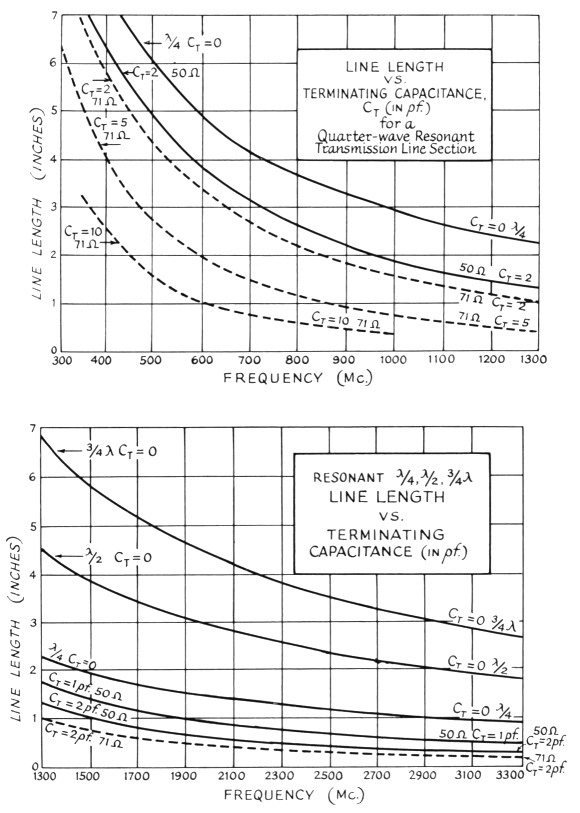Fig. 6. Nomograph for determining physical lengths of lines at various frequencies with relation to terminating capacitance.

These curves may be used for resonant lines having a characteristic impedance other than 71 ohms by using the conversion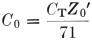where C0 is the terminating capacitance normalized with respect to the 71-ohm impedance.

To use this chart, determine the total minimum capacitance across the end of the line, including tube or tubes and tuning capacitor. Find the length of the line at the highest frequency used. Remember, the line can be lengthened electrically, or lowered in frequency by adding capacitance, but it can only be shorted electrically by cutting it off.

### Construction notes

The ideal way to build a coax-line amplifier or coaxial filter would be to use copper or brass tubing, silver plated on the conducting surfaces, and with all joints soldered. However, satisfactory results can be obtained with less effort. As an example, a coaxial filter for use on 6 and 2 meters was constructed, using a 3 × 4 × 17-inch aluminum chassis box and a 13½-inch length of 5/8-inch copper tubing. If 1-inch diameter tubing is used, a length of 14.12 inches should be about right. A 2¾ × 3¾-inch plate was soldered to one end of the tubing and mounted in the box. Input and output connectors were mounted on opposite sides and about 4 inches up from the base. Wire loops, the shape of an L, were spaced about 3 inch from the center conductor. A 3-30-pf. capacitor was connected halfway up the line. This provided enough capacitance to tune the line to resonance at 6 meters. The filter was tried on each band, with a power output of about 40 watts, into a wattmeter and 50-ohm load. The insertion loss was approximately 1 db. at center frequency. Spurious emissions and harmonics outside the bands should be suppressed by 40 to 50 db. Birdies and interference from TV and f.m. stations should also be similarly suppressed. When using a multiband antenna on 6 and 2, a filter such as this should help to prevent 6-meter third-harmonic energy from being radiated by the 2-meter section.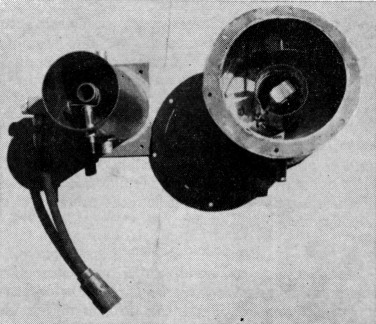Fig. 7. A photo of a typical 432-Mc. amplifier coaxial cavity (left), and a 432-Mc. coaxial filter, with crystal diode detector added, is shown at the right.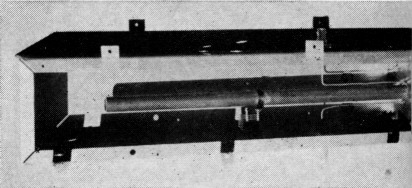A v.h.f. coaxial filter showing input and output coupling lines. The tuning capacitor is tapped down on the resonant element.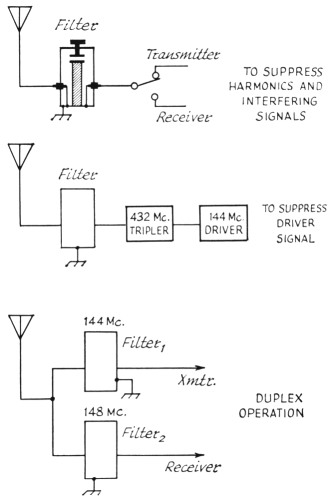Fig. 8. A block diagram illustrating three typical applications for coaxial filters.

### References

1. VHF Techniques, Vols. 1 and 2.
2. Radio Engineering Handbook, Ternman.
3. Radar Circuit Analysis, USAF.
4. "Lighthouse Tubes," General Electric ETX-110.
5. Moreno, Microwave Transmission Design Data, Dover Publications, Inc., New York, N. Y.
6. "Narrow Band Pre-selectors," Microlab Catalog No. 11A. Penfield, "Design of Quarter-Wave Resonant Lines," Electrical Design News, June, 1959.

### Notes

1. "The world above 50 Mc.," QST, February, 1961.
2. "A High-efficiency 2-meter kilowatt," QST, February, 1960.
3. Edinger, "A 4X250B amplifier for 144 Mc.," QST, October,1950.

Wilfred Jensby, WA6BQO.